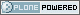Skip to content

# Neuroimaging Informatics Technology Initiative

##### Personal tools
You are here: Home » NIFTI1_INTENT_CODES

# NIFTI1_INTENT_CODES

nifti1 intent codes, to describe intended meaning of dataset contents. More...

## Defines

#define NIFTI_INTENT_CORREL   2
#define NIFTI_INTENT_TTEST   3
#define NIFTI_INTENT_FTEST   4
#define NIFTI_INTENT_ZSCORE   5
#define NIFTI_INTENT_CHISQ   6
#define NIFTI_INTENT_BETA   7
#define NIFTI_INTENT_BINOM   8
#define NIFTI_INTENT_GAMMA   9
#define NIFTI_INTENT_POISSON   10
#define NIFTI_INTENT_NORMAL   11
#define NIFTI_INTENT_FTEST_NONC   12
#define NIFTI_INTENT_CHISQ_NONC   13
#define NIFTI_INTENT_LOGISTIC   14
#define NIFTI_INTENT_LAPLACE   15
#define NIFTI_INTENT_UNIFORM   16
#define NIFTI_INTENT_TTEST_NONC   17
#define NIFTI_INTENT_WEIBULL   18
#define NIFTI_INTENT_CHI   19
#define NIFTI_INTENT_INVGAUSS   20
#define NIFTI_INTENT_EXTVAL   21
#define NIFTI_INTENT_PVAL   22
#define NIFTI_INTENT_LOGPVAL   23
#define NIFTI_INTENT_LOG10PVAL   24
#define NIFTI_FIRST_STATCODE   2
#define NIFTI_LAST_STATCODE   24
#define NIFTI_INTENT_ESTIMATE   1001
#define NIFTI_INTENT_LABEL   1002
#define NIFTI_INTENT_NEURONAME   1003
#define NIFTI_INTENT_GENMATRIX   1004
#define NIFTI_INTENT_SYMMATRIX   1005
#define NIFTI_INTENT_DISPVECT   1006
#define NIFTI_INTENT_VECTOR   1007
#define NIFTI_INTENT_POINTSET   1008
#define NIFTI_INTENT_TRIANGLE   1009
#define NIFTI_INTENT_QUATERNION   1010
#define NIFTI_INTENT_DIMLESS   1011

## Detailed Description

nifti1 intent codes, to describe intended meaning of dataset contents.

## Define Documentation

 #define NIFTI_FIRST_STATCODE   2
 Smallest intent_code that indicates a statistic.

 #define NIFTI_INTENT_BETA   7
 [C2, chap 25] Beta distribution (2 params): p1=a, p2=b. Density(x) proportional to x^(a-1) * (1-x)^(b-1).

 #define NIFTI_INTENT_BINOM   8
 [U, chap 3] Binomial distribution (2 params): p1 = number of trials, p2 = probability per trial. Prob(x) = (p1 choose x) * p2^x * (1-p2)^(p1-x), for x=0,1,...,p1.

 #define NIFTI_INTENT_CHI   19
 [C1, chap 18] Chi distribution (1 param): p1 = DOF. Density(x) proportional to x^(p1-1) * exp(-x^2/2) for x > 0. p1 = 1 = 'half normal' distribution p1 = 2 = Rayleigh distribution p1 = 3 = Maxwell-Boltzmann distribution.

 #define NIFTI_INTENT_CHISQ   6
 [C1, chap 18] Chi-squared (1 param): p1 = DOF. Density(x) proportional to exp(-x/2) * x^(p1/2-1).

 #define NIFTI_INTENT_CHISQ_NONC   13
 [C2, chap 29] Noncentral chi-squared statistic (2 params): p1 = DOF, p2 = noncentrality parameter.

 #define NIFTI_INTENT_DIMLESS   1011
 Dimensionless value - no params - although, as in _ESTIMATE the name of the parameter may be stored in intent_name.

 #define NIFTI_INTENT_DISPVECT   1006
 To signify that the vector value at each voxel is to be taken as a displacement field or vector: dataset must have a 5th dimensionintent_code must be NIFTI_INTENT_DISPVECTdim must be the dimensionality of the displacment vector (e.g., 3 for spatial displacement, 2 for in-plane)

 #define NIFTI_INTENT_ESTIMATE   1001
 To signify that the value at each voxel is an estimate of some parameter, set intent_code = NIFTI_INTENT_ESTIMATE. The name of the parameter may be stored in intent_name.

 #define NIFTI_INTENT_EXTVAL   21
 [C2, chap 22] Extreme value type I (2 params): p1 = location, p2 = scale cdf(x) = exp(-exp(-(x-p1)/p2)).

 #define NIFTI_INTENT_FTEST   4
 [C2, chap 27] Fisher F statistic (2 params): p1 = numerator DOF, p2 = denominator DOF.

 #define NIFTI_INTENT_FTEST_NONC   12
 [C2, chap 30] Noncentral F statistic (3 params): p1 = numerator DOF, p2 = denominator DOF, p3 = numerator noncentrality parameter.

 #define NIFTI_INTENT_GAMMA   9
 [C1, chap 17] Gamma distribution (2 params): p1 = shape, p2 = scale. Density(x) proportional to x^(p1-1) * exp(-p2*x).

 #define NIFTI_INTENT_GENMATRIX   1004
 To store an M x N matrix at each voxel: dataset must have a 5th dimension (dim=5 and dim>1)intent_code must be NIFTI_INTENT_GENMATRIXdim must be M*Nintent_p1 must be M (in float format)intent_p2 must be N (ditto)the matrix values A[i][[j] are stored in row-order: A A ... A[N-1]A A ... A[N-1]etc., untilA[M-1] A[M-1] ... A[M-1][N-1]

 #define NIFTI_INTENT_INVGAUSS   20
 [C1, chap 15] Inverse Gaussian (2 params): p1 = mu, p2 = lambda Density(x) proportional to exp(-p2*(x-p1)^2/(2*p1^2*x)) / x^3 for x > 0.

 #define NIFTI_INTENT_LABEL   1002
 To signify that the value at each voxel is an index into some set of labels, set intent_code = NIFTI_INTENT_LABEL. The filename with the labels may stored in aux_file.

 #define NIFTI_INTENT_LAPLACE   15
 [C2, chap 24] Laplace distribution (2 params): p1 = location, p2 = scale. Density(x) proportional to exp(-abs(x-p1)/p2).

 #define NIFTI_INTENT_LOG10PVAL   24
 Data is log10(p-value) (no params).

 #define NIFTI_INTENT_LOGISTIC   14
 [C2, chap 23] Logistic distribution (2 params): p1 = location, p2 = scale. Density(x) proportional to sech^2((x-p1)/(2*p2)).

 #define NIFTI_INTENT_LOGPVAL   23
 Data is ln(p-value) (no params).

 #define NIFTI_INTENT_NEURONAME   1003
 To signify that the value at each voxel is an index into the NeuroNames labels set, set intent_code = NIFTI_INTENT_NEURONAME.

 #define NIFTI_INTENT_NORMAL   11
 [C1, chap 13] Normal distribution (2 params): p1 = mean, p2 = standard deviation.

 #define NIFTI_INTENT_POINTSET   1008
 To signify that the vector value at each voxel is really a spatial coordinate (e.g., the vertices or nodes of a surface mesh): dataset must have a 5th dimensionintent_code must be NIFTI_INTENT_POINTSETdim = 5dim = number of pointsdim = dim = dim = 1dim must be the dimensionality of space (e.g., 3 => 3D space).intent_name may describe the object these points come from (e.g., "pial", "gray/white" , "EEG", "MEG").

 #define NIFTI_INTENT_POISSON   10
 [U, chap 4] Poisson distribution (1 param): p1 = mean. Prob(x) = exp(-p1) * p1^x / x! , for x=0,1,2,....

 #define NIFTI_INTENT_PVAL   22
 Data is a 'p-value' (no params).

 #define NIFTI_INTENT_QUATERNION   1010
 To signify that the vector value at each voxel is a quaternion: dataset must have a 5th dimensionintent_code must be NIFTI_INTENT_QUATERNIONdim = 5dim = 4datatype should be a floating point type

 #define NIFTI_INTENT_SYMMATRIX   1005
 To store an NxN symmetric matrix at each voxel: dataset must have a 5th dimensionintent_code must be NIFTI_INTENT_SYMMATRIXdim must be N*(N+1)/2intent_p1 must be N (in float format)the matrix values A[i][[j] are stored in row-order: AA AA A Aetc.: row-by-row

 #define NIFTI_INTENT_TRIANGLE   1009
 To signify that the vector value at each voxel is really a triple of indexes (e.g., forming a triangle) from a pointset dataset: dataset must have a 5th dimensionintent_code must be NIFTI_INTENT_TRIANGLEdim = 5dim = number of trianglesdim = dim = dim = 1dim = 3datatype should be an integer type (preferably DT_INT32)the data values are indexes (0,1,...) into a pointset dataset.

 #define NIFTI_INTENT_TTEST   3
 [C2, chap 28] Student t statistic (1 param): p1 = DOF.

 #define NIFTI_INTENT_TTEST_NONC   17
 [C2, chap 31] Noncentral t statistic (2 params): p1 = DOF, p2 = noncentrality parameter.

 #define NIFTI_INTENT_UNIFORM   16
 [C2, chap 26] Uniform distribution: p1 = lower end, p2 = upper end.

 #define NIFTI_INTENT_WEIBULL   18
 [C1, chap 21] Weibull distribution (3 params): p1 = location, p2 = scale, p3 = power. Density(x) proportional to ((x-p1)/p2)^(p3-1) * exp(-((x-p1)/p2)^p3) for x > p1.

 #define NIFTI_INTENT_ZSCORE   5
 [C1, chap 13] Standard normal (0 params): Density = N(0,1).

 #define NIFTI_LAST_STATCODE   24
 Largest intent_code that indicates a statistic.

Generated at Wed Mar 23 13:47:17 2005 for nifti1_io by1.2.8.1 written by Dimitri van Heesch, © 1997-2001
Created by Alex Clark
Last modified 2005-04-14 18:42This site conforms to the following standards: